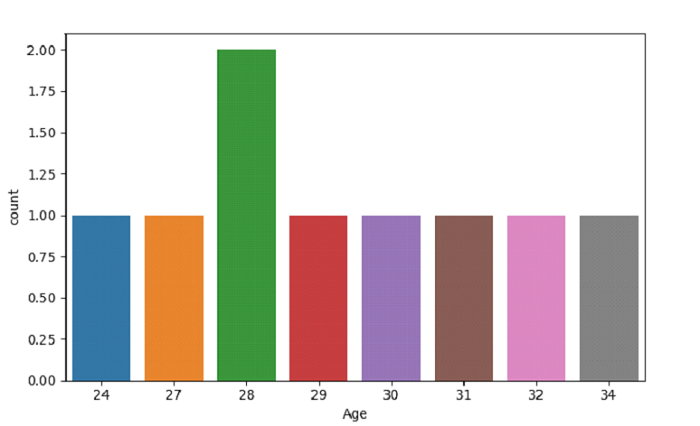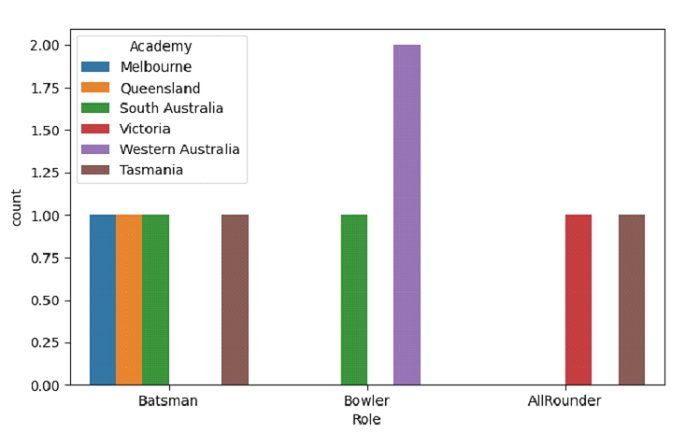# Create a Count Plot with SeaBorn â€“ Python Pandas

Count Plot in Seaborn is used to display the counts of observations in each categorical bin using bars. The seaborn.countplot() is used for this.

Let’s say the following is our dataset in the form of a CSV file − Cricketers.csv

At first, import the required 3 libraries −

import seaborn as sb
import pandas as pd
import matplotlib.pyplot as plt

Load data from a CSV file into a Pandas DataFrame −

dataFrame = pd.read_csv("C:\Users\amit_\Desktop\Cricketers.csv")


## Example

Following is the code −

import seaborn as sb
import pandas as pd
import matplotlib.pyplot as plt

# Load data from a CSV file into a Pandas DataFrame

# plotting count plot with Age column
sb.countplot(dataFrame["Age"])

# display
plt.show()

## Output

This will produce the following output −## Example

Let us see another example wherein we have used the hue parameter −

import seaborn as sb
import pandas as pd
import matplotlib.pyplot as plt

# Load data from a CSV file into a Pandas DataFrame

# plotting count plot with Role column and hue as Academy
plt.show()